RUSSIAN JOURNAL OF EARTH SCIENCES, VOL. 21, ES2004, doi:10.2205/2021ES000761, 2021

# Ice thickening caused by freezing of tidal jet

A. V. Marchenko1,2,3, E. G. Morozov4, A. V. Ivanov5, T. G. Elizarova5, D. I. Frey4

1Svalbard University Center, Longyearbyen, Spitsbergen, Norway

2Zubov State Oceanographic Institute, Moscow, Russia

3Sustainable Arctic Marine and Coastal Technology (SAMCoT)), Centre for Research-Based Innovation (CRI), Trondheim, Norway

4Shirshov Institute of Oceanology RAS, Moscow, Russia

5Keldysh Institute of Applied Mathematics, Moscow, Russia

### Abstract

We observed freezing of strong tidal jet of ice-free water as it flows under the ice in Lake Vallunden in the Van Mijen Fjord, Spitsbergen. The size of Lake Vallunden is approximately 1.2 km by 650 m, and its depth is 10 m. It is connected to the Van Mijen Fjord by a channel 100 long and 10 m wide. Due to strong tides, periodical tidal current in the channel exceeds 1 m/s. In winter, water temperature in the channel is close to freezing. It strongly cools while propagating along the ice-free channel. The jet of high velocity from the channel continues into the lake and its velocity decreases in the lake. As the strong current diverges and slows down in the lake, the water freezes in close vicinity of the channel. Ice thickness was measured over the entire lake. Intense freezing occurs approximately at a distance of 100 m from the channel where the velocity of the tidal jet decreases. The ice thickness in this region reaches 120 cm, whereas in the entire lake it is 70 cm. A mathematical model is suggested showing the velocity field of diverging and circulating tidal flow in the lake. The model for numerical simulation is based on a system of shallow water equations together with the transport equation.

### 1. Introduction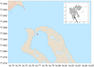Figure 1

Lake Vallunden is a lake in Spitsbergen approximately 1200 m long, its width is 600–650 m. The mean depth of the lake is 10–11 m. The lake is located near the shallow summit of the fjord at a distance of 55 km from the open ocean. The lake is connected to the Van Mijen Fjord by a channel 100 long and 10 m wide [Marchenko et al., 2013]. Strong tidal currents develop in this channel because the sea level in the fjord changes with the tidal periods. The amplitude of sea surface height in the fjord is approximately $\pm 1$ m. Due to strong tides, periodical tidal current in the channel exceeds 1 m/s. The lake and the fjord are covered with ice in winter, but the channel usually remains ice-free even in very cold winters because of the strong tidal flow in the channel. In winter, water temperature in the ice-free channel is close to freezing. Water strongly cools while propagating along the channel. The jet of high velocity from the channel continues into the lake and its velocity decreases in the lake approximately over a distance of 100 m. Field works were performed in the shallow area located in the end of the Van Mijen Fjord [Marchenko and Morozov, 2013, 2016a]. A chart of the region is shown in Figure 1. We study the continuation of the strong flow from the channel between the Van Mijen Fjord and Lake Vallunden near the Svea settlement (77° 53' N, 16° 46' E). In our previous studies [Marchenko and Morozov, 2013; Morozov et al., 2019] we observed a strong tidal flow in the channel connecting the Van Mijen Fjord and Lake Vallunden (and in Lake Vallunden). This tidal flow generates short period internal waves [Marchenko and Morozov, 2016b; Morozov and Pisarev, 2002; Morozov et al., 2019].

The goal of our research is to investigate the influence of very cold-water current on sea ice, which can cause changes in the ice thickness. The strong tidal currents in the channel continue into the lake preventing ice freezing along a narrow strip during relatively warm weather.

### 2. Experiment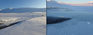Figure 2

Field works were performed in 2019 in Lake Vallunden (Spitsbergen) near the channel connecting the lake with the fjord. A narrow ($\sim$1–2 m wide) strip of water in the continuation of the flow from the strip does not freeze (Figure 2). We found an interesting phenomenon: ice thickness at the end of the ice-free strip appeared notably greater than in the lake. The mean ice thickness in the lake was approximately 70 cm.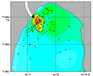Figure 3

To make a detailed map of the ice thickness near the channel in the northern part of the lake we performed a survey of ice thickness by drilling holes in the ice and measuring ice thickness with a graduated rod. The survey showed that the characteristic size of the region, in which thicker ice was found, is $\sim 100$ m. The region of thicker ice is located immediately near the end of the non-freezing tidal stream and around it. The maximum ice thickness in this region reaches 120 cm. At the border of this region, a significant gradient of ice thickness was found. Ice thickness sharply decreases over several meters to 80–90 cm and then gradually decreases to 70 cm, which is typical for the ice thickness throughout the lake (Figure 3).

We interpret this phenomenon as follows. When water flows into the lake along the non-freezing channel, the water temperature drops almost to the freezing point, and ice crystals and larger slash formations appear at the surface of the stream. This near-freezing water flows under the ice and ice crystals and slash join the ice sheet in the lake.

Strongly varying ice thickness near the strip was revealed by drilling measurements [Morozov et al., 2019]. Ice thickness near the ice-free strip sharply increased from 25 cm at the ice edge to 40–50 cm at about 1 m from the ice edge. At a distance of 5–8 m from the ice edge, the ice was as thick as 60 cm. In the continuation of the narrow strip, the ice thickness was almost 120 cm and then the thickness gradually decreased to about 70 cm in the entire lake. We explain the growth of ice thickness by the transport of small ice pieces and freezing water under the ice cover by the strong current and their accumulation under ice where the current speed decreases [Morozov et al., 2019].

### 3. Model

A system of regularized shallow water equations (RSWE) described in [Bulatov and Elizarova, 2011] has been used for numerical simulation of hydrodynamic processes in Lake Vallunden:

\begin{equation} \tag*{(1)} \frac{\partial h}{\partial t} + {\mathrm{div}} {\vec{j}}_m = 0, \end{equation} \begin{eqnarray*} \frac{\partial h\vec{u}}{\partial t} + {\mathrm{div}} \left( {\vec{j}}_m \otimes \ \vec{u}\right) + \vec{\nabla } \frac{gh^2}{2} = \end{eqnarray*}

\begin{equation} \tag*{(2)} h^* \left( \vec{f} - g\vec{\nabla} b \right) + {\mathrm{div}} \Pi , \end{equation}

\begin{equation} \tag*{(3)} h^* = h - \tau {\mathrm{div}} \left( h\vec{u} \right), \end{equation}

\begin{equation} \tag*{(4)} {\vec{j}}_m = h\left( \vec{u} - \vec{w} \right), \end{equation}

\begin{equation} \tag*{(5)} \vec{w} = \frac{\tau}{h} \left[ {\mathrm{div}} \left( h\vec{u} \otimes \vec{u} \right) + gh \vec{\nabla} \left(b + h \right) - h \vec{f} \right], \end{equation} \begin{eqnarray*} \Pi = \Pi_{\mathrm{NS}} + \tau \vec{u} \otimes \left[ h\left( \vec{u} \cdot \vec{\nabla} \right) \vec{u} + \right. \end{eqnarray*}

\begin{equation} \tag*{(6)} \left. gh\vec{\nabla} \left( b + h\right) - h\vec{f} \right] + \tau I\left[ gh\, {\mathrm{div}} \left( h\vec{u} \right) \right], \end{equation}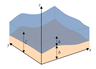Figure 4

where, $h(x,y,t)$ is the thickness of the water layer measured from the bottom, $b(x,y)$ is the bathymetry function; therefore, $\xi(x,y,t) = h(x,y,t) + b(x,y)$ is the level of water surface (Figure 4), $\vec{u} = \{u_x,u_y\}$ is the vector of horizontal velocity, $g=9.81$ m/s$^2$ is the acceleration due to gravity, $\vec{f} (x,y,t)$ is the vector of external volume force; $\tau > 0$ is regularization parameter, whose dimension is time. Besides, $\Pi_{\mathrm{NS}}$ is the Navier–Stokes viscous stress tensor, which, in a number of problems is considered as an additional regularizer and can be included or dropped, see, for example, [Bulatov and Elizarova, 2011; Sheretov, 2016].

The coefficient of kinematic viscosity of fluid $\mu$ is considered artificial; it is calculated from parameter $\tau$:

\begin{eqnarray*} \Pi_{NS} = \mu h \left[ \left( \vec{\nabla} \otimes \ \vec{u} \right) + \left( \vec{\nabla} \otimes \ \vec{u}\right)^{T} \right], \end{eqnarray*}

\begin{equation} \tag*{(7)} \mu =\tau gh. \end{equation}

Besides, a simplified model of the passive scalar transport is used to calculate the distribution of the inflowing cold water over the lake. Thus, the inflowing cold water is considered as an "impurity", where its concentration $C(x,y,t)$ could be proportional to the ice thickness. Concentration $C(x,y,t)$ is specified in dimensionless units. This model is based on the solution of the regularized transport equation:

\begin{eqnarray*} \frac{\partial Ch}{\partial t} + {\mathrm{div}} \left( {\vec{j}}_mC\right) = \end{eqnarray*}

\begin{equation} \tag*{(8)} {\mathrm{div}} \left( Dh\vec{\nabla}C + \tau \vec{u}h\left(\vec{u}\cdot \vec{\nabla }C\right)\right), \end{equation}

together with the RSWE system. The methods of deriving and solving the system of equations (1)–(8) are described in [Elizarova and Ivanov, 2020].

External volume force is represented by the Coriolis force

\begin{eqnarray*} \vec{f} = \left\{f_x,f_y\right\},\; f_x = f^c u_y, \; f_y = {-f}^cu_x. \end{eqnarray*}

The Coriolis force may be important because circular currents are formed in the lake and the Coriolis force can influence their formation. The Coriolis parameter is $f^c = 2\Omega \sin\varphi$, where $\Omega = 7.2921\times 10^{-5}$ s$^{-1}$ is the angular velocity of the Earth's rotation, which was assumed constant in the entire domain of simulations at latitude $\varphi = 77.87\mbox{°}$.

Before considering the model of bathymetry, let us describe the following designations: $\eta (x,y,t) = \xi(x,y,t)-\xi_0$ is the deviation of water level, where $\xi_0$ is the mean water level; $B(x,y) = b(x,y)-\xi_0$ is the depth of the lake measured from the mean level $\xi_0$.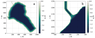Figure 5

Since the exact bathymetry data of Lake Vallunden has not been measured, an approximate bathymetry model, which is consistent with observations, has been developed, Figure 5. The shape of the lake was reproduced by transforming a satellite image, and the bathymetry was built on the assumption that the bottom 10-m deep is approximately flat, and the slopes at the coasts are very steep. In the northern part, there is an inlet channel, whose depth is 1.5 m, but there is a stone bar at the end of the channel where the depth reaches 1 m, Figure 5b. The width of the channel is approximately 10–15 m, the length is approximately 100 m. The height of the shores above the lake is 3–5 m.

Since the $y$-axis coincides with the direction of the normal to the boundary of the computational domain, the following boundary conditions were set to simulate the flow of cold water into the lake, at the boundary of the channel flowing into the lake ($x = 1250$ m, Figure 5b)

\begin{eqnarray*} \frac{\partial h}{\partial y} =0, \; \; u_y= -\sin \left( \frac{\pi t}{6}\right)(1-e^{-t/6}) {\mathrm{m/s}}, \end{eqnarray*} \begin{eqnarray*} u_x =0 {\mathrm{m/s}},\; C=1, \end{eqnarray*}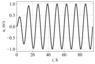Figure 6

where $t$ is given in hours. The profile of the normal velocity component at the channel boundary is shown in Figure 6.

The "dry bottom" condition was used at the other boundaries, as the boundary condition. The cutoff parameter $\varepsilon$ represents the minimum water level. Below this level, i.e., in the regions of the dry bottom, the liquid is at rest; therefore, a constraint is imposed on $\tau$ and $\vec{u}$ in the form

\begin{eqnarray*} \tau = \left\{ \begin{array}{c} \displaystyle{\frac{\alpha \sqrt{\Delta x \Delta y}} {\sqrt{gh}}},\; h > \varepsilon; 0, \qquad \qquad \; h \leq \varepsilon, \end{array} \right. \end{eqnarray*} \begin{eqnarray*} {\mathrm{if}} \: h< \varepsilon,\; {\mathrm{then}}\; h=\varepsilon \; {\mathrm{and}} \; \vec{u} =\vec {0}, \end{eqnarray*}

where $\Delta x, \Delta y$ are spatial grid steps. A similar problem without taking into account the propagation of impurities was considered using the system of regularized shallow water equations; its solution, as well as a description of the method for calculating the zones of flooding/drying are given in [Bulatov and Elizarova, 2016].

Since we consider cold water transport, it can be assumed that function $C$ is the temperature, and $D$ is the coefficient of thermal diffusivity of water. This coefficient is very small (at 0° C, $D \approx 13.2\times 10^{-8}$ m$^2$/s); therefore, it can be neglected. The currents in the lake are low (about 10 cm/s); therefore, the regularizer on the right-hand side of (8), represented by the dissipative term of the order of $\sim \tau hu^2$, is insufficient to ensure the stability of the numerical solution. That is why, in addition to the Navier–Stokes regularizer (7) in

equation (6), an artificial term $\delta \mu$ was introduced to coefficient $D$ in the transport equation (8) of the model, where $\delta$ is a dimensionless coefficient chosen from the conditions of stability and calculation accuracy, and $\mu$ is the viscosity coefficient taken from (7):

\begin{eqnarray*} D \rightarrow D +\delta \mu, \;\; \mu = \tau gh. \end{eqnarray*}

The first-order Euler scheme for time derivatives and second-order scheme for spatial derivatives were used for the numerical solution of the system of equations (1)–(8). A uniform rectangular grid with the following parameters was used:

\begin{eqnarray*} N_x=247, \; \Delta x = 4.1\; {\mathrm{m}}; \end{eqnarray*} \begin{eqnarray*} N_y=234, \; \Delta y = 5.4 \; {\mathrm{m}}; \end{eqnarray*} \begin{eqnarray*} \alpha = 0.3, \; \delta =0.1, \; \varepsilon =0.01 \;{\mathrm{m}}. \end{eqnarray*}

The time step was chosen in accordance with the Courant-Friedrichs-Lewy condition:

\begin{eqnarray*} \Delta t = \beta \frac{\left(\Delta x + \Delta y\right)} {2\sqrt{gh_{\max}}},\; \beta=0.2. \end{eqnarray*}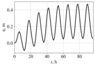Figure 7

We start modeling when the water is at rest. The inflow velocity is set (Figure 6) to gradually establish the water circulation in the lake. However, the periodical variations in the inflow velocity cause sea level fluctuations in the lake, which are established only from the 40-th model hour (Figure 7), and the mean water level increases up to about 20 cm. This can be explained by many factors. First, it should be noted that there are no drains in the lake, and its slopes are very steep. The narrow and shallow channel does not enable the water to completely flow from the lake during low tide. Part of the water remains in the lake due to which the total water level gradually increases during the first 50–60 model hours. Besides, during the numerical experiments, it was revealed that the shape and depth of the channel also play an important role. Various variants of the model approximations of the channel were considered in the simulations, for example, if we increased the width of the deep part of the channel, the water level in the lake changed with an amplitude of 1 m. The current view of the channel model agrees well with satellite images and experimental observations, and provides an amplitude of the water level change in the lake equal to $\pm 20$ cm, which is consistent with experimental observations.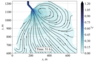Figure 8

As a result of several numerical experiments, it was found that the change in the mean water level almost did not have any effect on the circulation in the lake. The distribution of the concentration of coldest water is repeated with a periodicity of 12 h, which corresponds to the period of changes in the boundary conditions. A typical example of cold water propagation is shown in Figure 8. One can see a region with a radius of about 100 m and a larger region with a radius of about 300 m.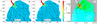Figure 9

Figure 9 shows the pattern of the flow and streamlines of the magnitude of velocity $|u| = \sqrt{u_x^2 + u_y^2}$ for the inflow (Figure 9a) and outflow (Figure 9b). The distribution of the velocity (of the order of several centimeters per second) far from the channel is consistent with the field measurements [Morozov et al., 2019]. A pattern of the formation of a circular cyclonic eddy with a diameter of 200–300 m is seen, which is also consistent with observations.

The concentration of the inflowing cold water determines the formation and distribution of ice thickness when the lake freezes. An eddy is formed in the solution. However, the form of the eddy and its relative location to the channel depends on small variations in the location of the channel and bottom topography near the channel. These variations almost do not influence the size of the eddy. The numerical experiments have shown that the presence of the Coriolis force does not affect the formation of circulation, i.e., circulation is caused solely due to the shape of the lake and the position of the inflow. The low influence of the Coriolis force is explained by the small spatial scale of the phenomenon. The effect of the Earth's rotation becomes important when the time scales of the motion are comparable with the inertial period. The size of the eddy in the lake is of the order of $\sim 100$ m and the velocity scale of motion in the lake is of the order of $\sim 0.1$ m/s. Hence, the time scale is of the order of $\sim 1000$ s, which is much smaller than the inertial period at latitude 78° N ($\sim 12$ h). Cold water is concentrated near the outflow from the channel to the lake (Figure 8). This corresponds to the real pattern of ice thickness distribution: ice is much thicker in the region of the eddy near the channel.

Let us compare the map of ice thickness in the inflow region with the circulation pattern. Figure 9 combines maps of ice thickness and currents in the lake, calculated using a numerical model. In the region of the lake, which is located close to the channel, the ice thickness is thicker, and a cyclonic eddy is formed by the inflowing tidal flow here. The size of the region of thick ice is the same as the region of high velocities near the channel as well as the size of the region of impurity (very cold water) in Figure 9a, Figure 9c.

### 4. Conclusions

Ice thickness in the region of the tidal jet flowing from the Van Mijen Fjord to Lake Vallunden was investigated in the winter season. The ice in the continuation of the ice-free strip is much thicker than in the lake. The ice-free continuation of the flow in the lake was about 2 m wide and 100 m long. Strong currents of almost freezing water penetrate under ice transporting ice crystals and slash, which attach to the ice cover from below, and thus ice thickness increases in the continuation of the flow. A numerical simulation allowed us to model the structure of currents in the lake when the tidal current flows into the lake. The flow initially forms an eddy which expands over the basin of the lake with decreasing velocities.

#### Acknowledgments

This field research was supported by the Norwegian Research Council (NFR) (project no. 196138/S30) and by the State Task of the Shirshov Institute of Oceanology (0128-2021-0002). Analysis of ice thickness was supported by the Russian Science Foundation (project no. 21-17-00278), model simulations were supported by the Russian Foundation for Basic Research (project nos. 19-57-60001 and 19-01-00262).

### References

Bulatov, O. V., T. G. Elizarova (2011) , Regularized shallow water equations and an efficient method for numerical simulation of shallow water flow, Computational Mathematics and Mathematical Physics, 51, no. 1, p. 160–173, https://doi.org/10.1134/S0965542511010052.

Bulatov, O. V., T. G. Elizarova (2016) , Regularized shallow water equations for numerical simulation of flows with a moving shoreline, Computational Mathematics and Mathematical Physics, 56, no. 4, p. 661–679, https://doi.org/10.1134/S0965542516040047.

Elizarova, T. G., A. V. Ivanov (2020) , Numerical modeling of passive scalar transport in shallow water based on the quasi-gasdynamic approach, Computational Mathematics and Mathematical Physics, 60, no. 7, p. 1208–1227, https://doi.org/10.1134/S0965542520070064.

Marchenko, A. V., E. G. Morozov (2013) , Asymmetric tide in Lake Vallunden (Spitsbergen), Nonlinear Processes in Geophysics, 20, p. 935–944, https://doi.org/10.5194/npg-20-935-2013.

Marchenko, A. V., E. G. Morozov (2016a) , Surface manifestations of the waves in the ocean covered with ice, Russ. J. Earth Sci., 16, p. ES1001, https://doi.org/10.2205/2016ES000561.

Marchenko, A. V., E. G. Morozov (2016b) , Seiche oscillations in Lake Vallunden (Spitsbergen), Russ. J. Earth. Sci., 16, p. ES2003, https://doi.org/10.2205/2016ES000567.

Marchenko, A. V., E. G. Morozov, S. V. Muzylev (2013) , Measurements of sea ice flexural stiffness by pressure characteristics of flexural-gravity waves, Annals of Glaciology, 54, p. 51–60, https://doi.org/10.3189/2013AoG64A075.

Morozov, E. G., S. V. Pisarev (2002) , Internal tides at the Arctic latitudes (numerical experiments), Oceanology, 42, no. 2, p. 153–161.

Morozov, E. G., A. V. Marchenko, K. V. Filchuk, et al. (2019) , Sea ice evolution and internal wave generation due to a tidal jet in a frozen sea, Applied Ocean Research, 87, p. 179–191, https://doi.org/10.1016/j.apor.2019.03.024.

Sheretov, Yu. V. (2016) , Regularized Hydrodynamic Equations, 222 pp., Tver State University, Tver, R.F (in Russian).

Received 25 February 2021; accepted 9 March 2021; published 9 April 2021.Citation: Marchenko A. V., E. G. Morozov, A. V. Ivanov, T. G. Elizarova, D. I. Frey (2021), Ice thickening caused by freezing of tidal jet, Russ. J. Earth Sci., 21, ES2004, doi:10.2205/2021ES000761.

Generated from LaTeX source by ELXfinal, v.2.0 software package.